## Group

A group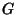is defined as a finite or infinite set of Operands (called elements''),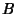,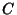, ... that may be combined or multiplied'' via a Binary Operator to form well-defined products and which furthermore satisfy the following conditions:

1. Closure: Ifandare two elements in, then the product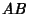is also in.

2. Associativity: The defined multiplication is associative, i.e., for all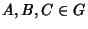,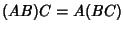.

3. Identity: There is an Identity Element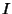(a.k.a.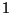,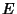, or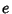) such that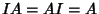for every element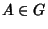.

4. Inverse: There must be an inverse or reciprocal of each element. Therefore, the set must contain an element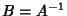such that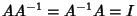for each element of.
A group is therefore a Monoid for which every element is invertible. A group must contain at least one element.

The study of groups is known as Group Theory. If there are a finite number of elements, the group is called a Finite Group and the number of elements is called the Order of the group.

Since each element,,, ...,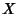, and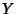is a member of the group, group property 1 requires that the product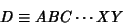(1)

must also be a member. Now apply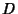to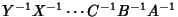,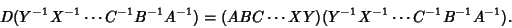(2)

But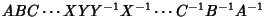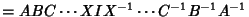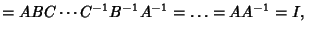(3)
so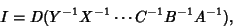(4)

which means that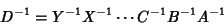(5)

and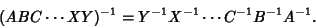(6)

An Irreducible Representation of a group is a representation for which there exists no Unitary Transformation which will transform the representation Matrix into block diagonal form. The Irreducible Representation has some remarkable properties. Let the Order of a Group be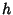, and the dimension of the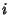th representation (the order of each constituent matrix) be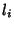(a Positive Integer). Let any operation be denoted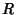, and let theth row andth column of the matrix corresponding to a matrixin theth Irreducible Representation be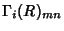. The following properties can be derived from the Group Orthogonality Theorem,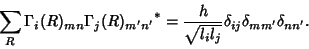(7)

1. The Dimensionality Theorem: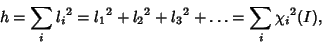(8)

where eachmust be a Positive Integer and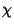is the Character (trace) of the representation.

2. The sum of the squares of the Characters in any Irreducible Representationequals,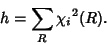(9)

3. Orthogonality of different representations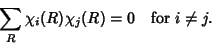(10)

4. In a given representation, reducible or irreducible, the Characters of all Matrices belonging to operations in the same class are identical (but differ from those in other representations).

5. The number of Irreducible Representations of a Group is equal to the number of Conjugacy Classes in the Group. This number is the dimension of the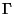Matrix (although some may have zero elements).

6. A one-dimensional representation with all 1s (totally symmetric) will always exist for any Group.

7. A 1-D representation for a Group with elements expressed as Matrices can be found by taking the Characters of the Matrices.

8. The number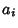of Irreducible Representations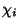present in a reducible representation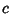is given by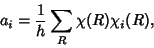(11)

whereis the Order of the Group and the sum must be taken over all elements in each class. Written explicitly,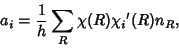(12)

where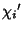is the Character of a single entry in the Character Table and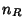is the number of elements in the corresponding Conjugacy Class.

See also Abelian Group, Adéle Group, Affine Group, Alternating Group, Artinian Group, Aschbacher's Component Theorem, Bp-Theorem, Baby Monster Group, Betti Group, Bimonster, Bordism Group, Braid Group, Brauer Group, Burnside Problem, Center (Group), Centralizer, Character (Group), Character (Multiplicative), Chevalley Groups, Classical Groups, Cobordism Group, Cohomotopy Group, Component, Conjugacy Class, Coset, Conway Groups, Coxeter Group, Cyclic Group, Dihedral Group, Dimensionality Theorem, Dynkin Diagram, Elliptic Group Modulo p, Engel's Theorem, Euclidean Group, Feit-Thompson Theorem, Finite Group, Fischer Groups, Fischer's Baby Monster Group, Fundamental Group, General Linear Group, General Orthogonal Group, General Unitary Group, Global C(G;T) Theorem, Groupoid, Group Orthogonality Theorem, Hall-Janko Group, Hamiltonian Group, Harada-Norton Group, Heisenberg Group, Held Group, Hermann-Mauguin Symbol, Higman-Sims Group, Homeomorphic Group, Hypergroup, Icosahedral Group, Irreducible Representation, Isomorphic Groups, Janko Groups, Jordan-Hölder Theorem, Kleinian Group, Kummer Group, Lp'-Balance Theorem, Lagrange's Group Theorem, Local Group Theory, Linear Group, Lyons Group, Mathieu Groups, Matrix Group, McLaughlin Group, Möbius Group, Modular Group, Modulo Multiplication Group, Monodromy Group, Monoid, Monster Group, Mulliken Symbols, Néron-Severi Group, Nilpotent Group, Noncommutative Group, Normal Subgroup, Normalizer, O'Nan Group, Octahedral Group, Order (Group), Orthogonal Group, Orthogonal Rotation Group, Outer Automorphism Group, p-Group, p'-Group, p-Layer, Point Groups, Positive Definite Function, Prime Group, Projective General Linear Group, Projective General Orthogonal Group, Projective General Unitary Group, Projective Special Linear Group, Projective Special Orthogonal Group, Projective Special Unitary Group, Projective Symplectic Group, Pseudogroup, Quasigroup, Quasisimple Group, Quasithin Theorem, Quasi-Unipotent Group, Representation, Residue Class, Rubik's Cube, Rudvalis Group, Schönflies Symbol, Schur Multiplier, Semisimple, Signalizer Functor Theorem, Selmer Group, Semigroup, Simple Group, Solvable Group, Space Groups, Special Linear Group, Special Orthogonal Group, Special Unitary Group, Sporadic Group, Stochastic Group, Strongly Embedded Theorem, Subgroup, Subnormal, Support, Suzuki Group, Symmetric Group, Symplectic Group, Tetrahedral Group, Thompson Group, Tightly Embedded, Tits Group, Triangular Symmetry Group, Twisted Chevalley Groups, Unimodular Group, Unipotent, Unitary Group, Viergruppe, von Dyck's Theorem

ReferencesGroup Theory

Arfken, G. Mathematical Methods for Physicists, 3rd ed. Orlando, FL: Academic Press, pp. 237-276, 1985.

Farmer, D. Groups and Symmetry. Providence, RI: Amer. Math. Soc., 1995.Weisstein, E. W. Groups.'' Mathematica notebook Groups.m.

Weyl, H. The Classical Groups: Their Invariants and Representations. Princeton, NJ: Princeton University Press, 1997.

Wybourne, B. G. Classical Groups for Physicists. New York: Wiley, 1974.